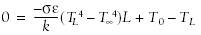# Using Excel to Help Solve Problems Formulated Using the Finite Element Method

Problem

You've applied the finite element method to a nonlinear problem and must now iteratively find a solution.

Solution

Use Solver to find a solution to the resulting nonlinear equation or system of equations. See Chapter 9 for recipes showing how to use Solver.

Discussion

The finite element method is a very popular method for numerically solving differential equations. The method involves discretizing the problem domain and governing equations, resulting in an algebraic system of equations that must be solved to arrive at an approximate solution to the problem. In some cases, the problem is nonlinear, which results in a nonlinear equation, or system of equations, that must be solved. As discussed in Chapter 9, Excel's built-in Solver tool is very adept at finding solutions to such equations.

By way of example, consider a heat conduction problem presented by Kythe and Wei in their Introduction to Linear and Nonlinear Finite Element Analysis.[*] The governing partial differential equation, with boundary conditions, is as follows:

[*] Prem Kythe and Dongming Wei, An Introduction to Linear and Nonlinear Finite Element Analysis (Boston: Birkhauser, 2004). See pages 244 and 245 for a complete derivation using the Galerkin method .This equation represents steady state heat conduction in a laterally insulated rod with constant temperature maintained at one end (x = 0) and with radiation heat transfer at the other end (x = L). k is the thermal conductivity of the rod material. s is the Stefan-Boltzman constant. [Image_Link]/images/U220A.jpg border=0> is the emissivity constant. Kythe and Wei apply the Galerkin method with linear shape functions to derive a solution for this problem. The resulting nonlinear equation to be solved is:TL represents the unknown temperature at the end of the rod. All other parameters in this equation are given. The solution to this equation was actually assigned as a homework problem in a graduate course I took with Dr. Wei. I used Excel's Solver tool to find a solution to this problem. Figure 12-11 shows the spreadsheet I prepared.

Cells C3 through C10 contain all of the given data for this problem. Cell C11 contains the unknown temperature at the end of the rod that must be found iteratively. Cell C12 contains the righthand side of the nonlinear equation shown earlier. In Figure 12-11, cell C12 is selected so that you can see the cell formula in Excel's formula bar. The formula is =-(C9*C10)/C6*(C11^4 - C8^4)*C5+C7-C11.

Figure 12-11. Solving for TL using SolverFigure 12-11 also shows the Solver window overlaid on the spreadsheet. The Solver model for this problem is relatively simple. Basically, you want to set the value of cell C12the one containing the nonlinear equationto 0 by changing the value in cell C11, which contains the temperature at the end of the rod. Setting up this model and pressing the Solve button in Solver yields the solution shown in Figure 12-11. That is, the temperature at the end of the rod is approximately 373.09 K.

Some approaches to formulating finite element solutions, for example the Rayleigh-Ritz approach, result in a minimization problem to find an approximate solution. In these cases, once the minimization problem has been formulated, you can use Excel's Solver tool. See Chapter 9 for more information on using Solver.

Solutions to many problems formulated using the finite element approach (particularly linear ones) result in an algebraic system of equations that must be solved. Here again, you can use techniques discussed in Chapter 9 to solve these systems.

Some finite element solution formulations require you to solve a system of ordinary differential equations in order to find an approximate solution to the original partial differential equation. Such systems can be solved in Excel. See Recipe 11.3 for an example.Excel Scientific and Engineering Cookbook (Cookbooks (OReilly))
ISBN: 0596008791
EAN: 2147483647
Year: N/A
Pages: 206
Authors: David M Bourg